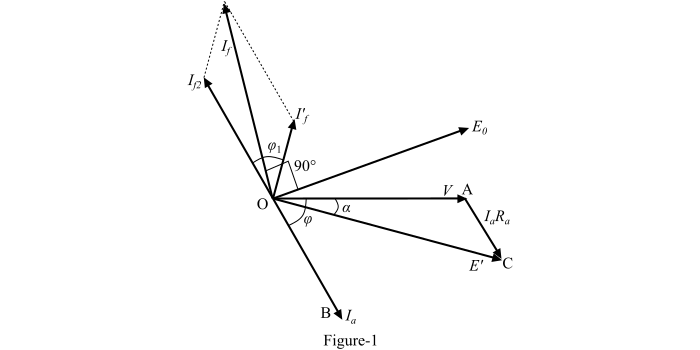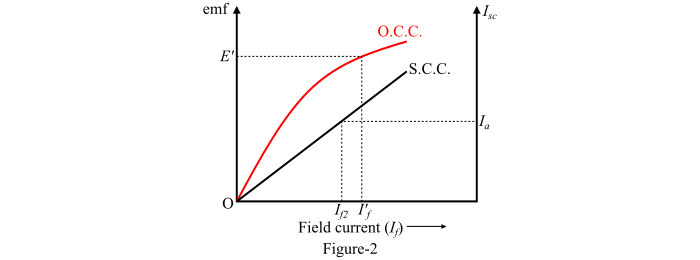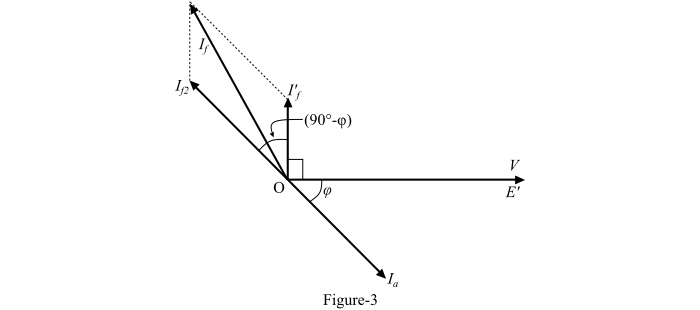# Voltage Regulation of Alternator by Ampere Turn Method

Electronics & ElectricalElectronDigital Electronics

#### Ethical Hacking & Cyber Security Online Training

36 Lectures 5 hours

#### Machine Learning & BIG Data Analytics: Microsoft AZURE

46 Lectures 3.5 hours

#### Advance Big Data Analytics using Hive & Sqoop

Best Seller

50 Lectures 4 hours

In the ampere-turn method (which is also known as the MMF method), the effect of armature leakage reactance is replaced by an equivalent additional armature reaction MMF so that this MMF can be combined with the armature reaction MMF.

In order to predict the voltage regulation of the alternator by the MMF method, the following information is required −

• Resistance of the armature (or stator) winding per phase.

• Open-circuit characteristic of the alternator.

• Short-circuit characteristic of the alternator.

The MMF method uses the phasor diagram of the alternator for determining the voltage regulation. In order to draw the phasor diagram at lagging power factor, the following procedure is used −

Step 1 – The armature terminal voltage per phase (V) is taken as the reference phasor along OA as shown in Figure-1.Step 2  – The armature current phasor (𝑰𝒂) is drawn lagging behind the voltage phasor (V) by a lagging power factor angle φ for which the voltage regulation of the alternator is to be calculated.

Step 3 – Draw the armature resistance drop phasor (𝑰𝒂𝑅𝒂) in phase with (𝑰𝒂) along the line AC. Now, join O and C and the phasor OC represents the EMF E’.Step 4 – From the open-circuit characteristic (O.C.C.) as shown in Figure-2, the field current (𝑰′𝒇) corresponding to the EMF (E’) is noted. Then, draw the phasor for the field current (𝑰′𝒇)) leading the EMF (E’) by 90°. It being assumed that under the short-circuit condition, all the excitation is opposed by the MMF of the armature reaction and armature reactance. Hence,

$$\mathrm{𝑰′_{𝒇} = 𝐼′_{𝑓}\angle(90° − α)}$$

Step 5 From the short-circuit characteristic (S.C.C.) also shown in Figure-2, determine the field current (𝑰𝒇𝟐) required to circulate the rated current on short-circuit. This is the field current required to overcome the synchronous reactance drop (𝑰𝒂𝑋𝑠). Thus, draw the phasor for the field current (𝑰𝒇𝟐) in phase opposition to the armature current (𝑰𝒂).

Step 6 – Now, in order to determine the resultant field current (𝑰𝒇), find the phasor sum of the field currents (𝑰′𝒇) and (𝑰𝒇𝟐). The current (𝑰𝒇) is the value of field current which would generate a voltage E0 under no-load conditions of the alternator.

Step 7 – Hence, the voltage regulation of the alternator can be determined from the expression given as follows −

$$\mathrm{Voltage\:Regulation =\frac{𝐸_{0} − 𝑉}{𝑉}× 100}$$

## Ampere-Turn Method with Ra Neglected

The phasor diagram of the alternator at lagging power factor with the armature resistance (Ra) of the alternator neglected is shown in Figure-3.Here,

$$\mathrm{𝑰_{𝑓2} = 𝐼_{𝑓2}\angle(180° − φ)}$$

And,

$$\mathrm{{{𝑰^{′}}_{𝒇}}= {{𝑰^{′}}_{𝒇}}\angle 90°}$$

Thus, the resultant field current is

$$\mathrm{𝑰_{𝑓} = {{𝑰^{′}}_{𝒇}} + 𝑰_{𝑓2}}$$